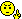# Graphing a parabola: only getting half of x = y^2 - 4

#### AGlas9837

##### Junior Member
I am trying to graph the parabola given by the equation x=y^2-4. Isolating y on one side I get y = sqrt(x+4). If I graph y = sqrt(x+4) I get the top half only of the parabola. How do I get the bottom half?! Am I entering the equation incorrectly?

#### stapel

##### Super Moderator
Staff member
Why are you doing this this hard way, trying to use your calculator? Are you absolutely required to, for some odd reason? :shock:

If you're allowed, just graph in the usual way, like you've always done: Pick input values (y-values, in this case), plug them into the equation, solve for the output values (x-values, in this case), draw the dots, and sketch the graph. The only difference here is that you're working "sideways" from the "normal" parabola, but the steps are otherwise exactly the same. You should not be "needing" your calculator for this!Eliz.

#### skeeter

##### Senior Member
AGlas9837 said:
I am trying to graph the parabola given by the equation x=y^2-4. Isolating y on one side I get y = sqrt(x+4). If I graph y = sqrt(x+4) I get the top half only of the parabola. How do I get the bottom half?! Am I entering the equation incorrectly?
$$\displaystyle y = \sqrt{x+4}$$ is the top "half" of the parabola (the positive side)

$$\displaystyle y = -\sqrt{x+4}$$ will give you the bottom half (the negative side)

in function mode, the calculator cannot graph both at the same time using only one function (the entire parabola is not a function, correct?) ... so, put the expression for the positive side in Y1 and the one for the negative side in Y2 ... the calculator graphs two distinct functions and gives you the graph of the "whole" parabola sitting on its side.